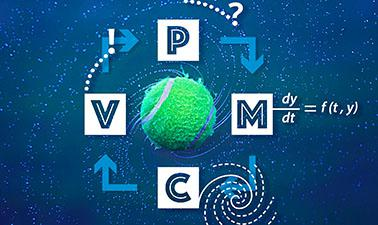# Delft University of Technology: Modelling with Differential Equations

How do populations grow? How do viruses spread? What is the trajectory of a glider? Introduce yourself to the modelling cycle which includes: analyzing a problem, formulating it as a mathematical model, calculating solutions and validating your results.6 weeks
4–5 hours per week
Self-paced
Free

All models are (systems of) ordinary differential equations. In this course you will learn more about those by watching video lectures and reading short texts, and more importantly, by completing well-crafted hands-on exercises in which you can practice modeling yourself!

You will also learn how to implement Euler's method in a (Python) program.

In the verified track of this course you will additionally consolidate the new skills with graded problem sets about four other real-life applications.

This course is aimed at Bachelor students from Mathematics, Engineering and Science disciplines. However it is for anyone who would want to use differential equations for solving real-world problems, including business owners, researchers and students.

### At a glance

• Institution: DelftX
• Subject: Math
• Level: Intermediate
• Prerequisites:

Calculus (1st-order ordinary differential equations), some programming skills are helpful. This course is aimed at Bachelor students from Mathematics, Engineering and Science disciplines.

• Language: English
• Video Transcript: English
• Associated skills: Differential Equations, LaTeX, Mathematical Modeling, Python (Programming Language)

# What you'll learn

Skip What you'll learn
• To follow the process of the mathematical modelling cycle: formulate a real-life problem, construct an appropriate mathematical model, calculate solutions and validate the results.
• Analyze and use (systems of) ordinary differential equations.
• Solve the ordinary differential equations and implement Euler's method in a (Python) program.

In the Verified Track, you will additionally:

• Complete well-crafted problem sets on several interesting real-life applications to consolidate your new skills

# Syllabus

Skip Syllabus

Module 1:

Introduction to the cycle of mathematical modelling. We will start describing a population of fish by a differential equation.

Verified Track : Two practice problems (filtering with sunscreen, mixing fluids) with other real-life applications to consolidate the theory learned.

Module 2:

Complete more modelling cycles by improving on the model and evaluating the consequences. Euler's method is introduced for solving ordinary differential equations. You will run Python simulations.

Verified Track : A new application to practice the theory: the spread of a flu epidemic.

Module 3:

Predator fish are added to the model. How do the populations interact? Systems of differential equations.

Verified Track : One more practice problem (electrical clock) to consolidate the theory learned about systems.

# Learner testimonials

Skip Learner testimonials

"Why do I need mathematics is no longer a question for me. So many phenomena and problems can be modelled using mathematics. I really enjoyed making a model to describe how the virus we studied spread. Every engineering or science student should take this course!"

"This course is excellent! I am an engineer, but have been working in another field for almost 2 decades, totally away from calculus, and this is exactly what I was looking for in order to brush up. I loved the videos and the questions as well. They are crafted in a very clever way to sediment concepts just learned."